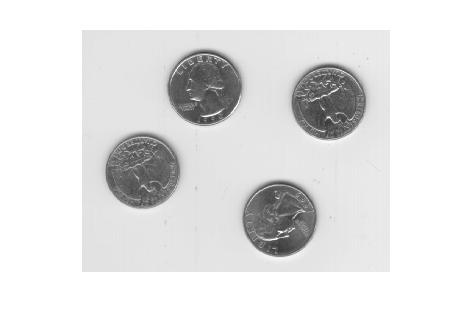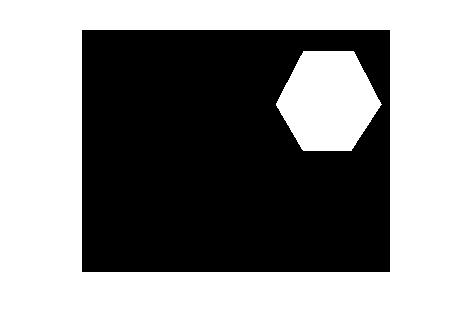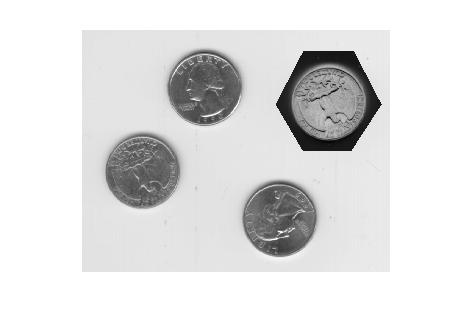# ROIPOLY – rectangular regions and logical indexing

Several readers commented on my recent roipoly and poly2mask posts, and I wanted to follow up on a couple of issues they raised. The first issue is a common point of confusion about the selection of rectangular regions, and the second is how to access and modify pixels contained within a region of interest.

### Rectangular regions

Users sometimes wonder why poly2mask and roipoly don't return some of the boundary pixels of a rectangular region as part of the mask. Here's an example.

x = [2 4 4 2 2];
y = [2 2 4 4 2];

mask = poly2mask(x, y, 5, 5)
mask =

0     0     0     0     0
0     0     0     0     0
0     0     1     1     0
0     0     1     1     0
0     0     0     0     0



Some users expect rows 2 through 4 and columns 2 through 4 to be included in the mask. One reader commented that he uses a different function instead of roipoly because of this behavior.

As I have illustrated in my previous posts, roipoly and poly2mask treat a pixel not as a point, but as a unit square area. The upper-left corner of the (2,2) pixel is (1.5,1.5), and the lower-right corner is (2.5,2.5). So the polygon defined by the x-y vertices in the example above covers only one-fourth of the (2,2) pixel.

I suggest that you get used to defining rectangles that cover complete pixel areas. Such rectangles go along pixel edges, not through pixel centers. Here's a second example to demonstrate:

xp = [1.5 4.5 4.5 1.5 1.5];
yp = [1.5 1.5 4.5 4.5 1.5];

maskp = poly2mask(xp, yp, 5, 5)
maskp =

0     0     0     0     0
0     1     1     1     0
0     1     1     1     0
0     1     1     1     0
0     0     0     0     0



Now the "mystery" is completely removed about which border rows and columns are in the mask. There's no need to use some other function.

### Logical indexing

Another reader asked how to get and modify pixels inside a region of interest. One nice way to do that in MATLAB is to use logical indexing. The expression A(B), if B is logical and the same size as A, selects all the elements in A corresponding to the true elements of B.

I'll demonstrate using an example mask from the roipoly documentation.

clear
imshow(I)c = [222 272 300 270 221 194];
r = [21 21 75 121 121 75];
imshow(mask)If you use whos or look in the workspace browser, you can see that mask is a logical matrix.

whos
  Name        Size             Bytes  Class      Attributes

I         242x308            74536  uint8
c           1x6                 48  double
r           1x6                 48  double



The expression I(mask) is a column vector containing all the elements of I corresponding to the true values of mask. Here, for example, is how you would compute the mean of the pixels inside the region of interest:

mean(I(mask))
ans =

156.0468



You can use logical indexing on the left-hand side of an assignment as well.

I2 = I;
imshow(I2)Here's a documentation link for logical indexing. Or you might be interested in this MATLAB Digest article that Loren and I wrote about MATLAB indexing.

Published with MATLAB® 7.3

|